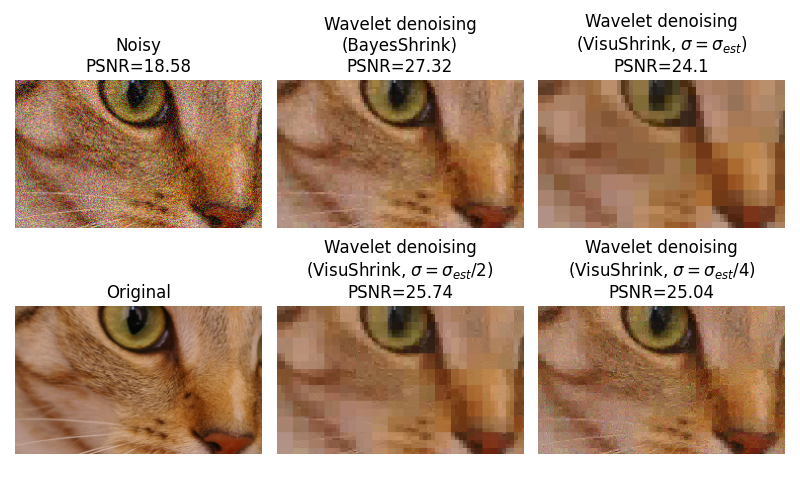# Wavelet denoising#

Wavelet denoising relies on the wavelet representation of the image. Gaussian noise tends to be represented by small values in the wavelet domain and can be removed by setting coefficients below a given threshold to zero (hard thresholding) or shrinking all coefficients toward zero by a given amount (soft thresholding).

In this example, we illustrate two different methods for wavelet coefficient threshold selection: BayesShrink and VisuShrink.

## VisuShrink#

The VisuShrink approach employs a single, universal threshold to all wavelet detail coefficients. This threshold is designed to remove additive Gaussian noise with high probability, which tends to result in overly smooth image appearance. By specifying a sigma that is smaller than the true noise standard deviation, a more visually agreeable result can be obtained.

## BayesShrink#

The BayesShrink algorithm is an adaptive approach to wavelet soft thresholding where a unique threshold is estimated for each wavelet subband. This generally results in an improvement over what can be obtained with a single threshold.Estimated Gaussian noise standard deviation = 0.11716133039704142
Clipping input data to the valid range for imshow with RGB data ([0..1] for floats or [0..255] for integers).
Clipping input data to the valid range for imshow with RGB data ([0..1] for floats or [0..255] for integers).
Clipping input data to the valid range for imshow with RGB data ([0..1] for floats or [0..255] for integers).


import matplotlib.pyplot as plt

from skimage.restoration import (denoise_wavelet, estimate_sigma)
from skimage import data, img_as_float
from skimage.util import random_noise
from skimage.metrics import peak_signal_noise_ratio

original = img_as_float(data.chelsea()[100:250, 50:300])

sigma = 0.12
noisy = random_noise(original, var=sigma**2)

fig, ax = plt.subplots(nrows=2, ncols=3, figsize=(8, 5),
sharex=True, sharey=True)

plt.gray()

# Estimate the average noise standard deviation across color channels.
sigma_est = estimate_sigma(noisy, channel_axis=-1, average_sigmas=True)
# Due to clipping in random_noise, the estimate will be a bit smaller than the
# specified sigma.
print(f'Estimated Gaussian noise standard deviation = {sigma_est}')

im_bayes = denoise_wavelet(noisy, channel_axis=-1, convert2ycbcr=True,
method='BayesShrink', mode='soft',
rescale_sigma=True)
im_visushrink = denoise_wavelet(noisy, channel_axis=-1, convert2ycbcr=True,
method='VisuShrink', mode='soft',
sigma=sigma_est, rescale_sigma=True)

# VisuShrink is designed to eliminate noise with high probability, but this
# results in a visually over-smooth appearance.  Repeat, specifying a reduction
# in the threshold by factors of 2 and 4.
im_visushrink2 = denoise_wavelet(noisy, channel_axis=-1, convert2ycbcr=True,
method='VisuShrink', mode='soft',
sigma=sigma_est/2, rescale_sigma=True)
im_visushrink4 = denoise_wavelet(noisy, channel_axis=-1, convert2ycbcr=True,
method='VisuShrink', mode='soft',
sigma=sigma_est/4, rescale_sigma=True)

# Compute PSNR as an indication of image quality
psnr_noisy = peak_signal_noise_ratio(original, noisy)
psnr_bayes = peak_signal_noise_ratio(original, im_bayes)
psnr_visushrink = peak_signal_noise_ratio(original, im_visushrink)
psnr_visushrink2 = peak_signal_noise_ratio(original, im_visushrink2)
psnr_visushrink4 = peak_signal_noise_ratio(original, im_visushrink4)

ax[0, 0].imshow(noisy)
ax[0, 0].axis('off')
ax[0, 0].set_title(f'Noisy\nPSNR={psnr_noisy:0.4g}')
ax[0, 1].imshow(im_bayes)
ax[0, 1].axis('off')
ax[0, 1].set_title(
f'Wavelet denoising\n(BayesShrink)\nPSNR={psnr_bayes:0.4g}')
ax[0, 2].imshow(im_visushrink)
ax[0, 2].axis('off')
ax[0, 2].set_title(
'Wavelet denoising\n(VisuShrink, $\\sigma=\\sigma_{est}$)\n'
'PSNR=%0.4g' % psnr_visushrink)
ax[1, 0].imshow(original)
ax[1, 0].axis('off')
ax[1, 0].set_title('Original')
ax[1, 1].imshow(im_visushrink2)
ax[1, 1].axis('off')
ax[1, 1].set_title(
'Wavelet denoising\n(VisuShrink, $\\sigma=\\sigma_{est}/2$)\n'
'PSNR=%0.4g' % psnr_visushrink2)
ax[1, 2].imshow(im_visushrink4)
ax[1, 2].axis('off')
ax[1, 2].set_title(
'Wavelet denoising\n(VisuShrink, $\\sigma=\\sigma_{est}/4$)\n'
'PSNR=%0.4g' % psnr_visushrink4)
fig.tight_layout()

plt.show()


Total running time of the script: ( 0 minutes 0.900 seconds)

Gallery generated by Sphinx-Gallery